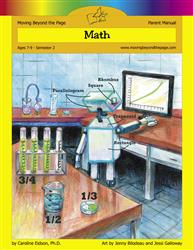# Common Core Alignment

CCSS.Math.Content.3.G.2 - Partition shapes into parts with equal areas. Express the area of each part as a unit fraction of the whole. For example, partition a shape into 4 parts with equal area, and describe the area of each part as 1/4 of the area of the shape.

## 5: MathUnit 7: Geometry
Lesson 6: Dividing Shapes
Lesson 7: Some Geometric Problem Solving
Lesson 8: Unit Test